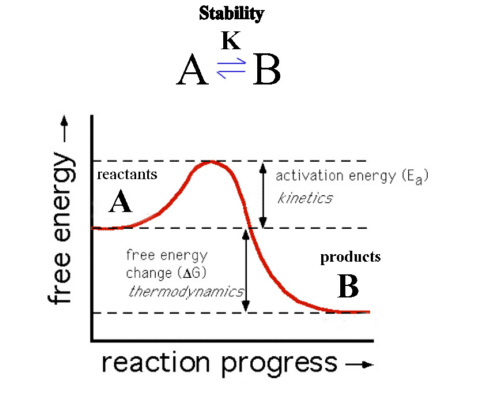## Stability

When discussing the concept of Stability it is necessary to distinguish between thermodynamic and kinetic stability.Here B is at lower energy than A so that ΔG is negative. The reaction should therefore proceed spontaneously and B is the more thermodynamically stable species.

The reaction as shown though has a barrier to the progress of the reaction called the Activation Barrier (Ea) and so the reaction may proceed very slowly. The thermodynamics describes only the starting and ending position of the reaction and not the intermediate or transition state. If the kinetics is slow, A is described as being inert while if it proceeds quickly then A is described as being labile.

The conditions that distinguish them are:
if the reaction takes longer than 1 minute under the conditions of concentration 0.1 M, temperature 25°C, then it is INERT,
if under the same conditions the reaction time < 1 minute, then it is LABILE.

In the lecture on isomerism, we depend on the samples being kinetically stable i.e. inert.

In the lecture on Chelation and Stability we concentrate on thermodynamic stability and look at the changes in free energy, enthalpy and entropy during the reaction.
When we consider thermodynamic stability we need to be familiar with 2 formulae:
ΔG = - RT ln(K) or alternatively ΔG = - 2.303RT log10(K) ---(1)
ΔG = ΔH - TΔS ---(2)

The first relates the free energy to the stability constant and the second shows the breakdown into the component enthalpy and entropy terms.Return to Chemistry, UWI-Mona, Home Page